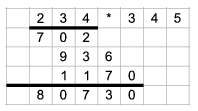Mathematical and Physical Journal
for High Schools
Issued by the MATFUND Foundation
 Already signed up? New to KöMaL?

#Problem I. 111. (September 2005)

I. 111. Let us demonstrate long multiplication by multiplying two 3-digit numbers.

Prepare an Excel sheet so that one can enter two integers with 3 digits (each digit in a separate cell) into a specific place in the first row. The next few rows should contain the progress and the result of the long multiplication of these numbers. You can assume that the numbers in fact have 3 digits, so, for example, no first digit can be 0.The sheet (i111.xls) is to be submitted.

(10 pont)

Deadline expired on October 17, 2005.

Solution. A possible solution is here.

### Statistics:

 40 students sent a solution. 10 points: Balambér Dávid, Bóra Eszter, Bőle Pál, Czigler András, Dancsó Péter, Deák Tibor, Fábián András, Fejér Attila, Gál Tibor, Gilián Zoltán, Gombkötő Tamás, Gulyás 990 Péter, Györök Péter, Horváth 151 Gábor, Kisfaludi-Bak Sándor, Kiss Dániel Miklós, Kovács 129 Péter, Ozsvárt László, Ökrös Tamás, Szoldatics András, Varga 842 Iván, Varga Attila József, Vecsey Dénes, Véges Márton, Vincze János. 8 points: 10 students. 6 points: 2 students. 2 points: 1 student. 0 point: 2 students.

Problems in Information Technology of KöMaL, September 2005